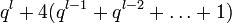# Element structure of special linear group of degree two over a finite discrete valuation ring

## Contents

This article gives specific information, namely, element structure, about a family of groups, namely: special linear group of degree two. This article restricts attention to the case where the underlying ring is a finite discrete valuation ring.
View element structure of group families | View other specific information about special linear group of degree two | View other specific information about group families for rings of the type finite discrete valuation ring

This article discusses the element structure of the special linear group of degree two over a finite discrete valuation ring (i.e., local principal ideal ring) with residue field having size$q$, underlying prime characteristic of residue field$p$, and length$l$. The size of the whole ring is$q^l$, and each successive power of the unique maximal ideal has index$q$ in its predecessor.

Examples are$\mathbb{Z}/(p^l\mathbb{Z})$ (here$p = q$),$\mathbb{F}_q[t]/(t^l)$, and Galois rings.

The Galois ring with residue field of size$q$ and length$l$ is the unique (up to isomorphism) discrete valuation ring obtained as a degree$\log_pq$ extension of$\mathbb{Z}/p^l\mathbb{Z}$, and hence generalizes both$\mathbb{Z}/(p^l\mathbb{Z})$ and$\mathbb{F}_q$. The Galois ring has characteristic$p^l$.

## Summary

Item Value
order of the group$q^{3l - 2}(q^2 - 1)$.
number of conjugacy classes Case$q$ odd:$q^l + 4 \frac{q^l - 1}{q - 1}$, which is$q^l + 4(q^{l-1} + q^{l-2} + \dots + 1)$
Case$q$ a power of 2: PLACEHOLDER FOR INFORMATION TO BE FILLED IN: [SHOW MORE]